×

Search anything:

# Stack vs Queue [Differences]

#### Algorithms Stack queueGet this book -> Problems on Array: For Interviews and Competitive Programming

Contents:

• What is a Stack?
• What is a Queue?
• Differences between stack and queue.
• Similarities between stack and queue.
• Implementation.

## What is a Stack?

A stack is a linear data structure in which both insertion and deletion operation occurs at one end. It is based on LIFO (last-in-first-out) principle in which both insertion and deletion takes place from one end only. So, stack is basically a container which is closed from one end. In case of an array, we can access any element of an array at any time, whereas in a stack, we can only access the elements of the stack in the sequential manner. In stack,w e can only insert elements of same data type.

Operations Performed on a Stack:

1. push(x) : It is used to insert an element to the top of the stack.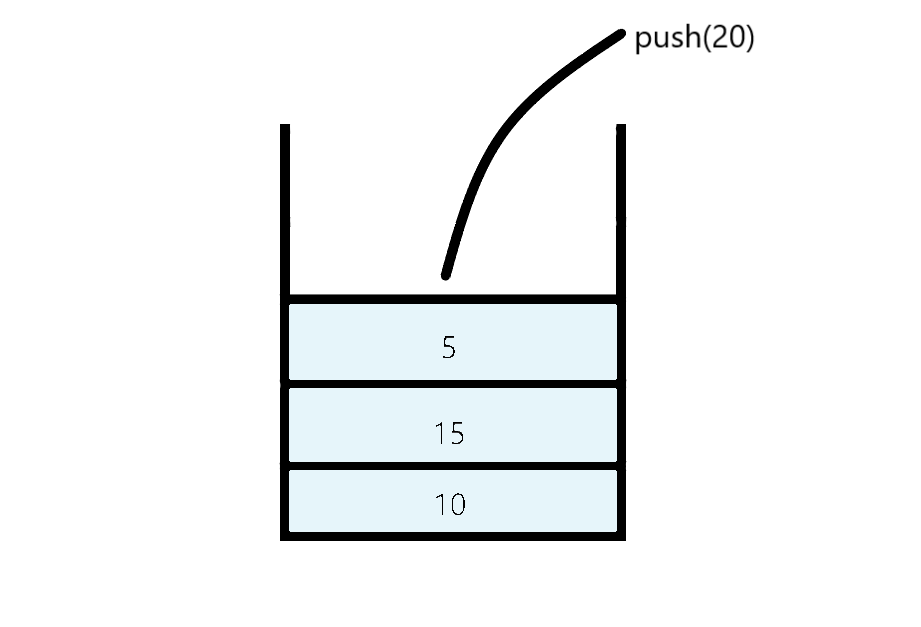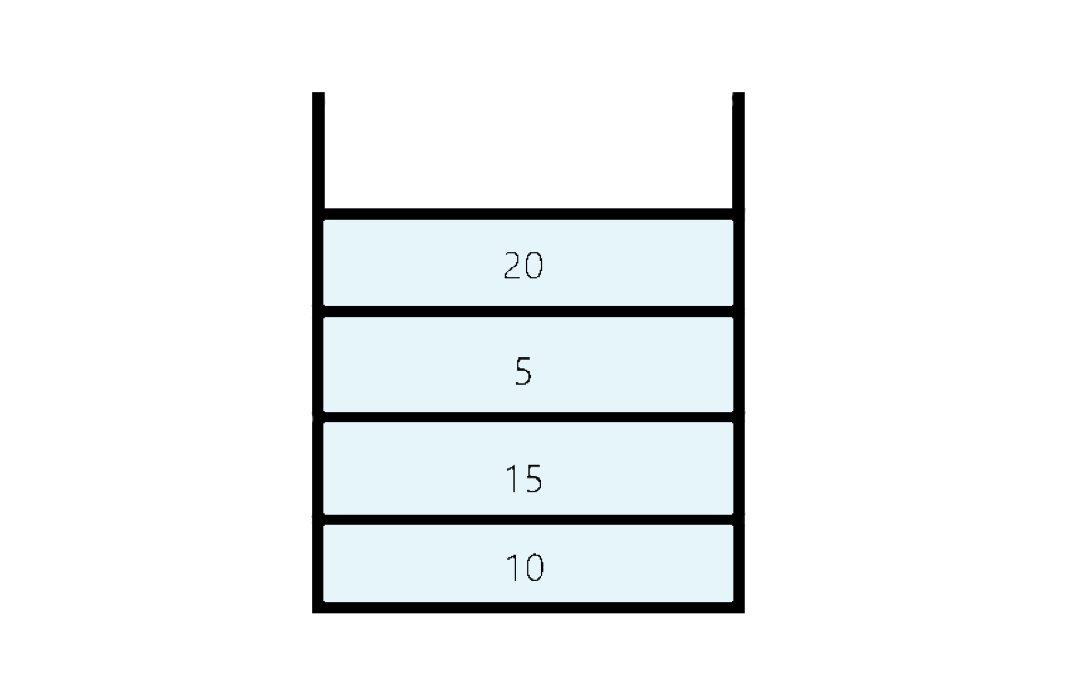2. pop() : It removes the element from the top and returns it value.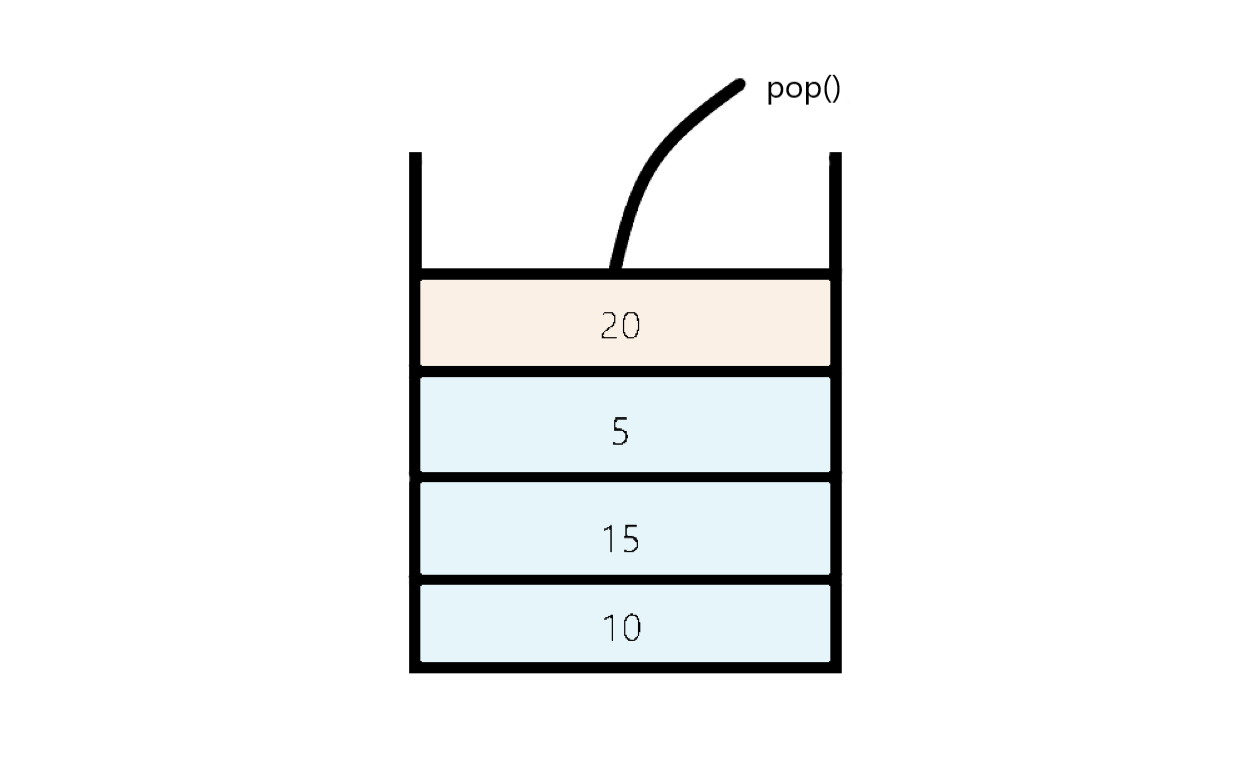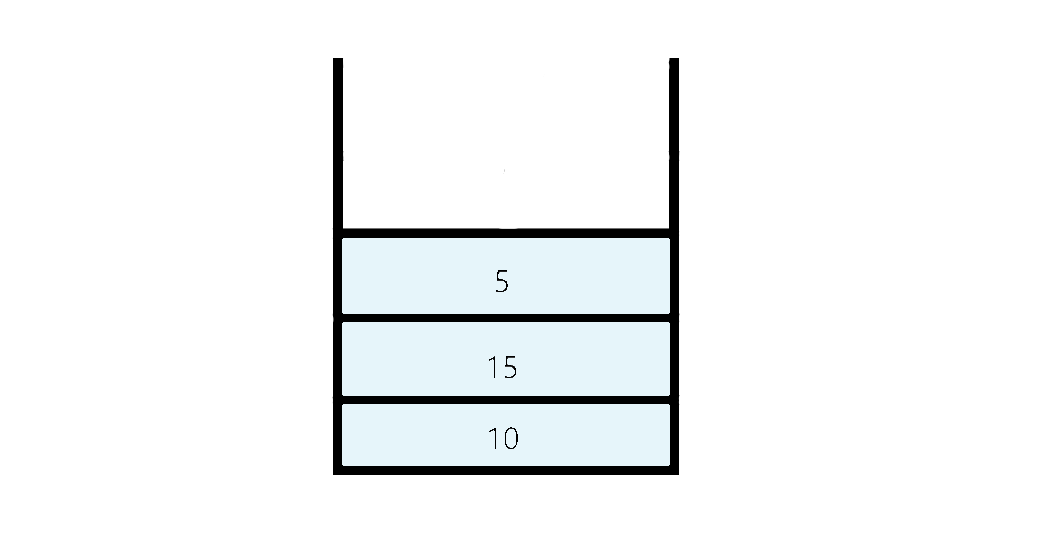3. peek() : It returns the element present at the top.
4. size() : It returns the current size of the size.
5. isEmpty() : Returns true if stack is empty i.e, if there is no elements present in the stack.

## What is a Queue?

Queue is a linear data structure which is based on first in first out (FIFO) principle i.e, the element which have been inserted first will be removed first.
unlike stacks queue is open from two ends in which insertion from one end and deletion from the other end. we can imagine queue similar to the queue of people at a ticket counter in which first person at the queue will get the ticket and will get out from the queue. The end from which the insertion happens in queue is known as REAR where as, the end from which the deletion happens is known as FRONT.

Operations Performed on a Queue:

1. enqueue() : It is used to add or insert an item to the queue.
2. dequeue() : It is used to remove an item from the queue and return it.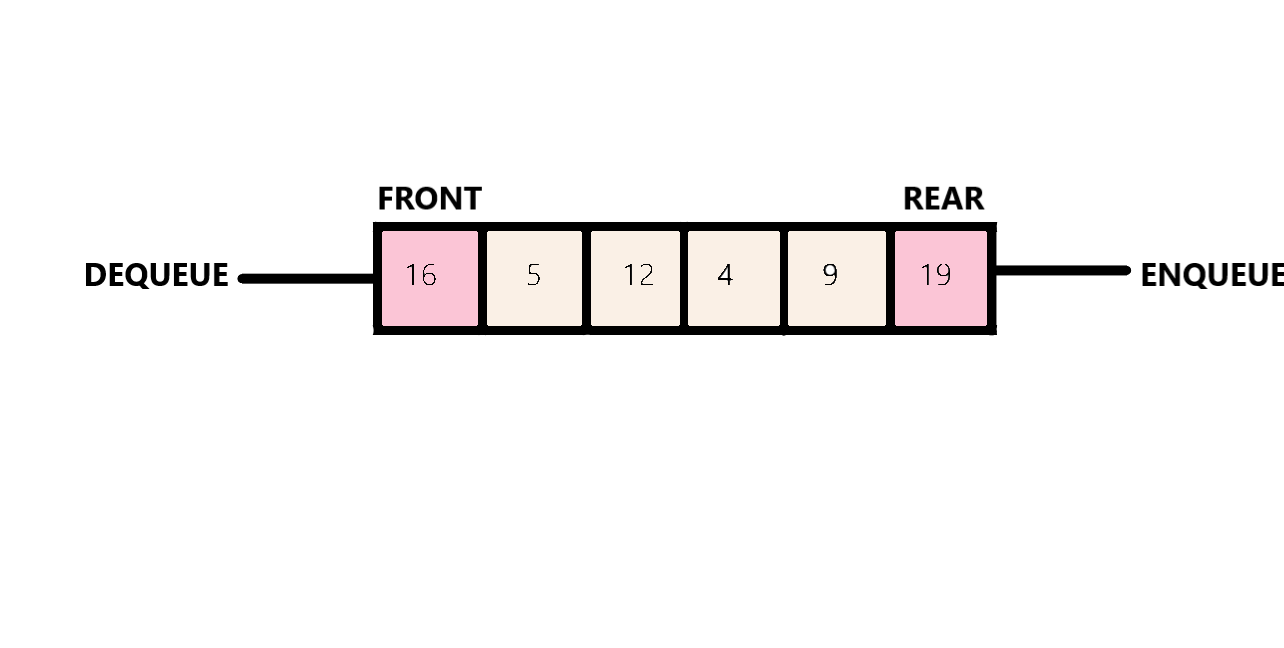3. getFront() : It returns the front element of the queue from where deletion happens.
4. getRear() : It returns the rear element of the queue from where insertion happens.
5. size() : It returns the size of the queue.

## Differences between stack and queue.

1. Stack follows LIFO (Last in First Out) principle in which the element which has been inserted at last will be removed first.
Where as, Queue follows FIFO (First in First Out) principle in which the element which has been inserted at first will be removed first.

2. Stack is open from one end only from which both insertion and deletion happens which is also known as top.
Where as, Queue are open from both ends. The end from where insertion happens is known as rear amd the other end from where deletion occurs is known as front.

3. Stack mainly performs two operations push and pop. The push operation is used to add an element in the stack and the pop operation is used to remove the element from the stack.
Where as, Queue also performs mainly two operation enqueue and dequeue. The enqueue operation is used to insert an element in the queue while the dequeue operation removes the element from the queue.

4. Stack is considered as full if If top== max-1.
Where as, Queue is considered as full If rear==max-1.

5. Stack is considered as empty if If top== -1.
Where as, Queue is considered as full If If front== -1 or front = rear+1

6. Stacks are visualized as vertical collections.
Where as, Queues are visualized as horizontal collections.

## Similarities between stack and queue.

1. Both Stack and queue are linear data structure i.e, the elements in them are stored sequentially.
2. Both of them are flexible in size and can grow according to requirement of input.
3. Both stack and queue can be implemented by using Array and Linked list.

## Implementation.

Here I am going to implement stack and Queue using Linked list as it is the most efficient way to implement stack as well as queue.

``````import java.io.*;
import java.util.*;

class Node{
int data;
Node next;
Node(int d){
data=d;
next=null;
}
}

class MyStack{
int size;
MyStack(){
size=0;
}

void push(int x){
Node temp=new Node(x);
size++;
}

int pop(){
{
return Integer.MAX_VALUE;
}
size--;
return res;
}

int peek(){
{
return Integer.MAX_VALUE;
}
}

int size(){
return size;
}

boolean isEmpty(){
}
}

class Test{

public static void main (String[] args)
{
MyStack s=new MyStack();
s.push(15);
s.push(10);
s.push(25);
System.out.println(s.pop());
System.out.println(s.size());
System.out.println(s.peek());
System.out.println(s.isEmpty());
}
}

``````

``````import java.util.*;
import java.io.*;
import java.lang.*;
class Node {
int val;
Node next;

public Node(int val)
{
this.val = val;
this.next = null;
}
}

class Queue {
Node front, rear;

public Queue()
{
this.front = this.rear = null;
}

void enqueue(int val)
{

Node temp = new Node(val);

if (this.rear == null) {
this.front = this.rear = temp;
return;
}

this.rear.next = temp;
this.rear = temp;
}

void dequeue()
{

if (this.front == null)
return;

Node temp = this.front;
this.front = this.front.next;

if (this.front == null)
this.rear = null;
}
}

public class Test {
public static void main(String[] args)
{
Queue q = new Queue();
q.enqueue(10);
q.enqueue(20);
q.dequeue();
q.dequeue();
q.enqueue(30);
q.enqueue(40);
q.enqueue(50);
q.dequeue();
System.out.println("Queue Front : " + q.front.val);
System.out.println("Queue Rear : " + q.rear.val);
}
}
``````

The main difference in the dynamic implementation of stack vs queue are:

1. In stack we define only one pointer "head" pointing to the top element of the stack, where as in Queue we define two pointers "front" and "rear" pointing to the first and last element of the queue respectively.
2. While inserting element in the stack we make the head pointer point to that element that we are inserting where as, in queue we make the rear pointer points to that element that we are inserting.
3. While removing a element from stack we make the head pointer point to the head.next where as, In Queue we make front pointer point to front.next.

#### Uddeshya Raj

Uddeshya Raj is pursuing B. Tech in Computer Science from Chandigarh University. He is an Algorithm and Data Structure Developer, Intern at OpenGenus.